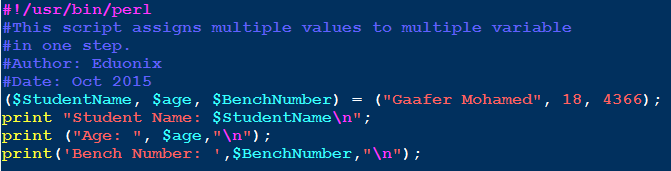Perl ProgrammingLearn about Scalar Variable in Perl Programming Language

# Learn about Scalar Variable in Perl Programming Language

In the previous article, we had a short introduction to Variables. The first of the three main types of variables supported by the Perl language is Scalar Variables.

Scalar variables are the normal ordinary variables supported by all programming languages. Other languages call them just variables. A scalar variable can hold a single piece of data at a time. This value could be a number (either integer, float, octal, or hexadecimal) or a string of text.

Scalar variable names are Perl identifiers preceded with dollar sign ‘\$’. The dollar sign can be considered as part of the scalar variable name, that the variable couldn’t be recognized as a variable without.

Scalar Variable Assignment
To assign a value for a scalar variable, the assignment operator ‘=’ is used.

Examples

```\$name = "Mohamed Ali";
\$e = 2.718;			# The base of the natural logarithm
\$Counter = 3;
\$ch = 'A';
```

Multiple Variables Assignment
Perl allows assigning different values to multiple scalar variables in one step. This uses the following syntax:

`(\$VAR1,\$VAR2,…,\$VARn) = (VALUE1,VALUE2,…,VALUEn);`

Examples
The following script will assign multiple values to multiple variables.After performing the assignment step, it prints the values of the three variables.The statement:

`(\$StudentName, \$age, \$BenchNumber) = ("Gaafer Mohamed", 18, 4366);`

is short for the following three assignment statement:

```\$StudentName = "Gaafer Mohamed";
\$age = 18;
\$BenchNumber = 4366;
```

Although the typing is almost the same in both cases, the multiple assignment statement keeps your code short (don’t take this nine-lines short script as a measure. Someday, you may get involved in writing a Perl program with 5 or 10 thousands lines).

Multiple Variables Assignment to the Same Value
Consider the case wherein you need to initialize several scalar variables to the same value. For instance, we are writing a script that makes a sorting operation and some calculations. We need to define a loop counter, a variable that stores the grand total, and a variable to be used for swapping values of two variables. The logic of the script dictates initializing all these variables to zero. We can simply go ahead and type the following lines:

```\$counter  = 0;
\$grandtotal = 0;
\$swap = 0;
```

– Aha, you won’t deceive me this time. You want to say that we can better do it as follows:

`(\$counter, \$grandtotal, \$swap) = (0, 0, 0);`

Right?!

Mmm, yes… and no.

Yes, your way is correct, and does the job in a compact form. But, no, that is not what I meant. Look at this new way:

`\$counter = \$grandtotal = \$swap = 0;`

Isn’t it more beautiful?!

Multi-line Strings
Strings can be as short as these:

```\$empty = "";
\$ch = 'A';
\$name = "Julia Smith"
```

Or as long as the as this:

```\$longstr = "First they ignore you,
then they laugh at you,
then they fight you,
then you win."
```

Perl allows assigning multi-line strings to scalar variables.Effect of Single & Double Quotes on Embedded Scalar Variables
As we have seen in the previous article, double quotes preserve the meaning of escape sequences like \n (new line character), \t (Tap), etc. On the other hands, single quotes don’t preserve the meaning for their content. So, \n is printed \n (not new line).

This rule remains true with variables.
So, if we have a scalar variable \$name whose value is “Mohamed Ali”, the output in both cases will be as follows:

```print ("Name: \$name");

Name: Mohamed Ali
```

Per contra, single quotes will have another opinion:

```print ('Name: \$name');

Name: \$name
```

* * * * * *

Conclusions

• In this article, we have talked about the first type of variables that Perl supports: the Scalar Variables.
• A scalar variable can hold a single piece of data at a time. Integer, float, octal, hexadecimal and string values, all could be possible values for scalar variables.
• Scalar variable names are Perl identifiers preceded with a dollar sign ‘\$’.
• Assignment of values to scalar variables is done using the assignment operator ‘=’
• While double quotes print the values of variables correctly as expected, single quotes don’t.

I hope you find this article useful. See you in the next article: Operators.

### Why Is Design Thinking Important In Digital Transformation?

21,501Fans
4,210Followers
104,000Subscribers

### Why Is Design Thinking Important In Digital Transformation?

- Advertisement -